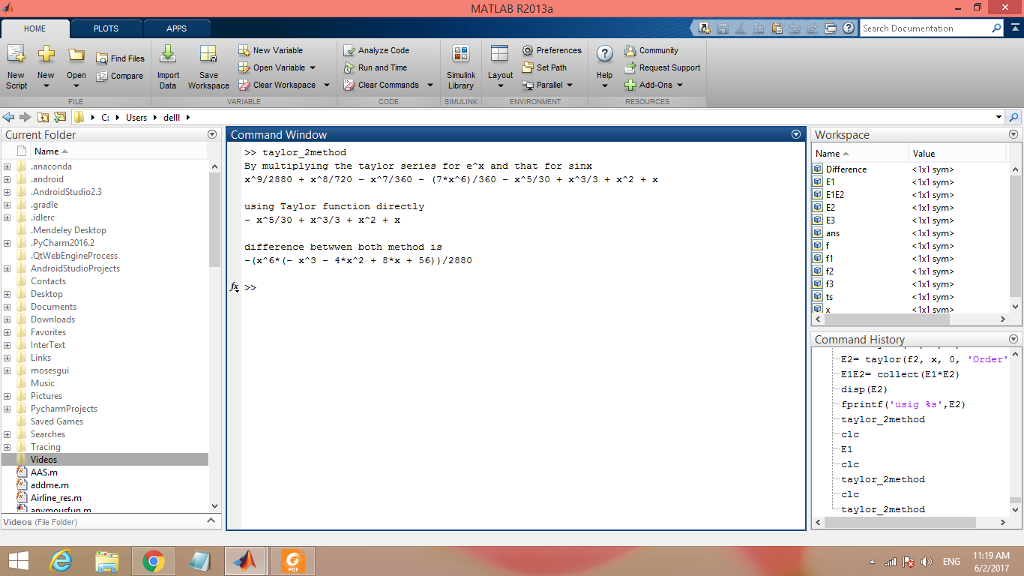# Answered! Matlab Question Find the taylor series for e^x sin x about x=0 in two ways: (a) By multiplying the taylor series for e^x and that for sinx….

Matlab Question

Find the taylor series for e^x sin x about x=0 in two ways:

Don't use plagiarized sources. Get Your Custom Essay on
Answered! Matlab Question Find the taylor series for e^x sin x about x=0 in two ways: (a) By multiplying the taylor series for e^x and that for sinx….
GET AN ESSAY WRITTEN FOR YOU FROM AS LOW AS \$13/PAGE

(a) By multiplying the taylor series for e^x and that for sinx.

(b) By using the taylor function directly on e^x sin x.

I found this solution, but I’m having trouble implementing it. Can someone break it down or make it simplier for me (what suppose to be in a scrpit file, what suppose to be in the command window, etc.), or if you have a different way of solving it please share. Thanks in advance.

syms x

E1= taylor(exp(x), 5);

E2= taylor(sin(x), 6);

E1E2= collect(E1*E2)

E3= collect(taylor(exp(x)*sin(x), 6))

Difference= simplify(E1E2 – E3)

E1E2=

E3=

x+x^2+1/3*x^3-1/30*x^5

Difference=

1/2880*x^9+1/720*x^8-1/360*x^7-7/260*x^6

code to type in script file. i have named script fie as taylor_2method.m

—————————————————————————————————————————————————-

%Taylor serier find using two methods in one script

syms x;

f1 = exp(x);

f2=sin(x);
f3=exp(x)*sin(x);

% taylor function use 5 five parameter.
% 3rd parameter is the point around which the expansion is made.
% 5th parameter is order that is n+1. if we are giving order 5 , it will
% give term start from 4th degree polynonimal (x^4……..).

%E1 Find taylor series of exp(x).
E1= taylor(f1, x, 0, ‘Order’, 5);

%E2 Find taylor series of sin(x)
E2= taylor(f2, x, 0, ‘Order’, 6);

%E3 Find taylor series of exp(x)*sin(x)
E3= taylor(f3, x, 0, ‘Order’, 6);

E1E2= collect(E1*E2);
Difference= simplify(E1E2 – E3);

disp(‘By multiplying the taylor series for e^x and that for sinx’);
disp(E1E2);

disp(‘using Taylor function directly’);
disp(E3);

disp(‘difference betwwen both method is’);
disp(Difference);
————————————————————————————————————————————————–

Then simply run this script .Output will be shown to commnad window. Output contains tayor series by using both methods as mentioned in question as well as difference between the output of both methods.

Output